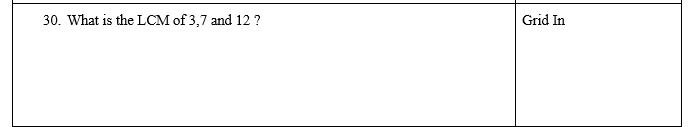SHSAT Math CW#02 Number Sense Part 2
SHSAT Classwork Assignment (30 Questions)
Email *
Question #1: Simplify a Fraction
1 point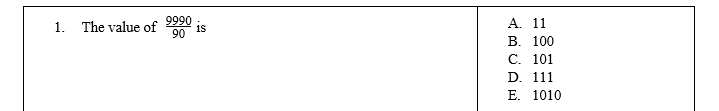Question #2: Three Fraction Terms
1 point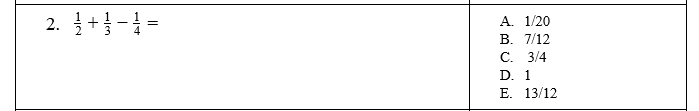Question #3: Equivalent Exponent
1 point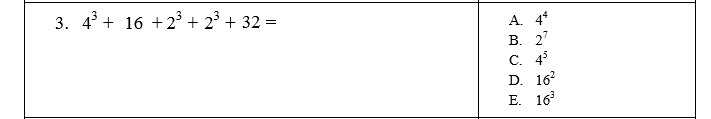Question #4: Repeating Decimals and Fractions
1 point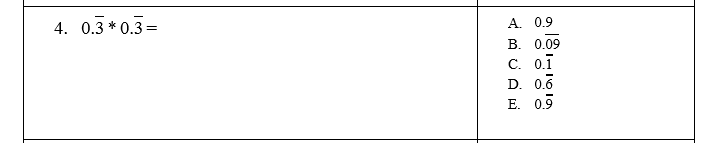Question #5: Closest to 1.
1 point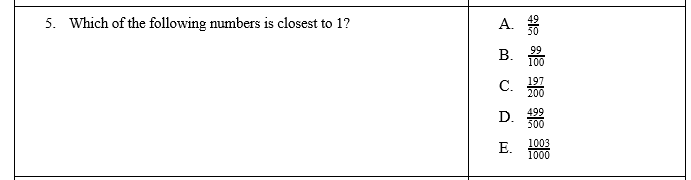Question #6: Compare Inequalities
1 point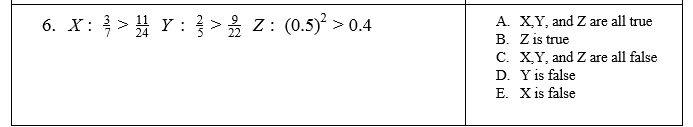Question #7: Average of Sets
1 point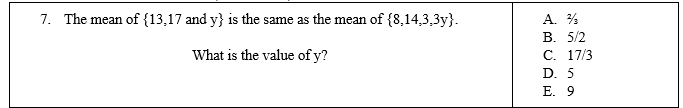Question #8: Convert to Decimal
1 point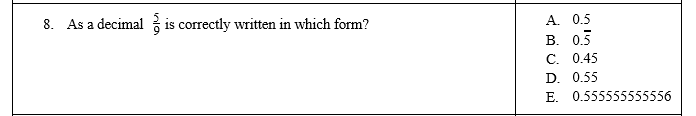Question #9: Solve for Variable "n"
1 point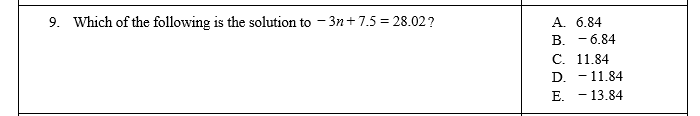Question #10: Equivalent Expression
1 point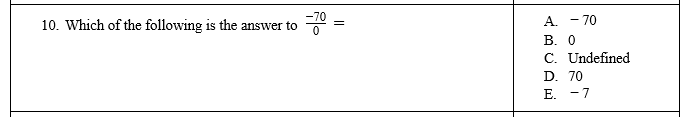Question #11: Properties of Mathematics
1 point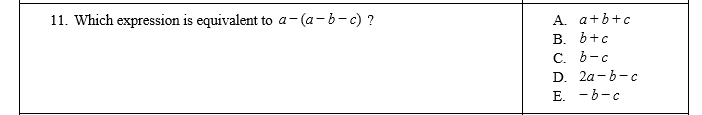Question #12: Even & Odd Expressions
1 point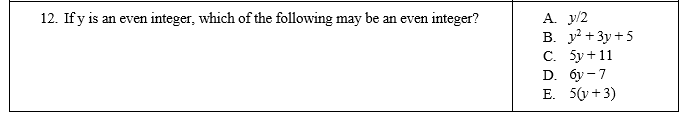Question #13: Comparing Inequalities
1 point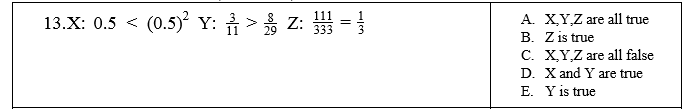Question #14: Closest to a given value.
1 point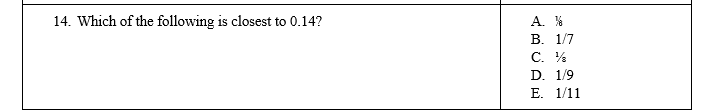Question #15: Weekly Calendar
1 point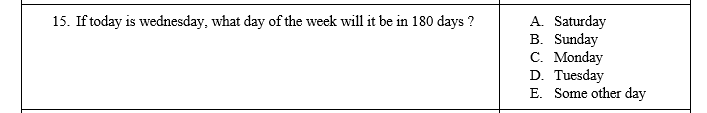Question #16: Closest to a Stated Value
1 point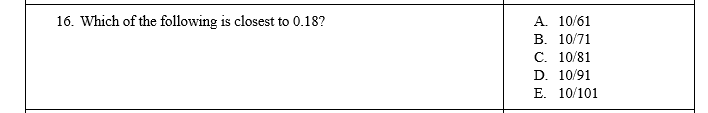Question #17: Use Estimation with Square Roots
1 pointQuestion #18: Triangles are 180 Degrees
1 point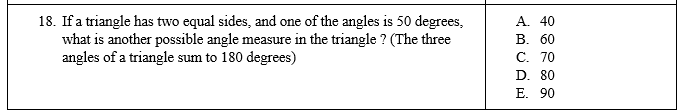Question #19: Absolute Value with 2 Variables
1 point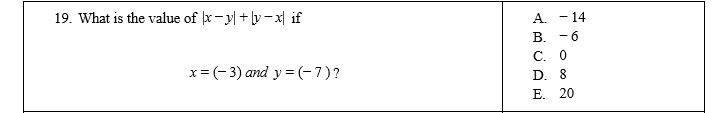Question #20: Prime Number Theory
1 point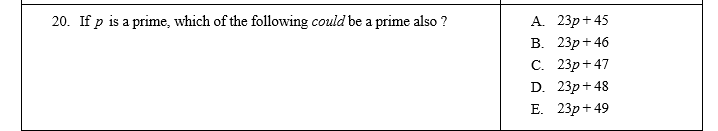Question #21: Working with Different Denominators
1 point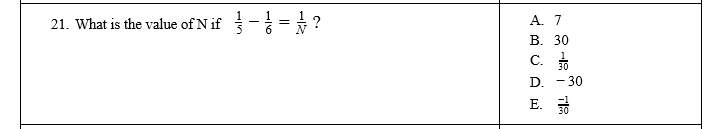Question #22: Midpoints (P=-10)(Q=-5)(R=1)(S=7)
1 point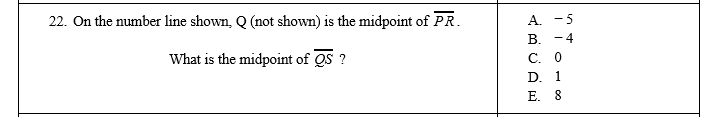Question #23: Absolute Value Calculation
1 point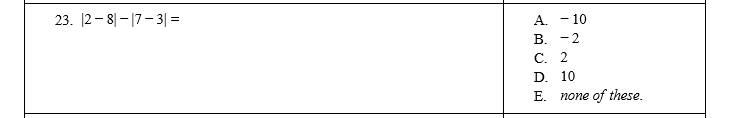Question #24: Exponents and Prime Factors
1 point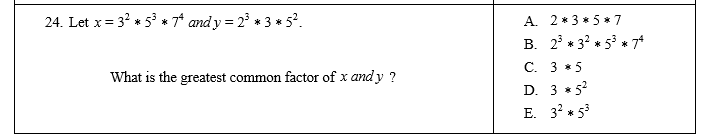Question #25: Factorials (Example: 4! = 4*3*2*1=24)
1 point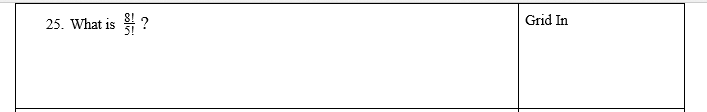Question #26: "Twin" Primes
1 point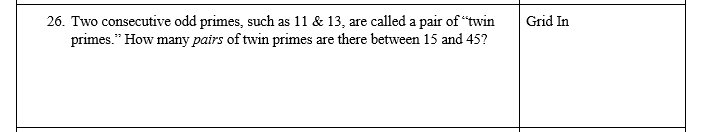Question #27: Express in Decimal Form
1 point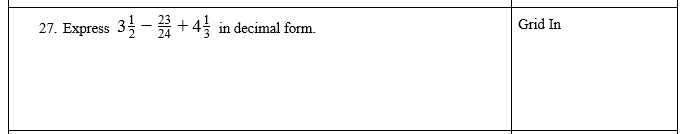Question #28: Solve for an Exponent Value
1 point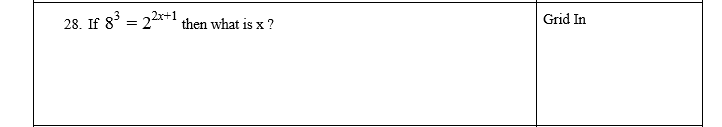Question #29: Smallest Value
1 point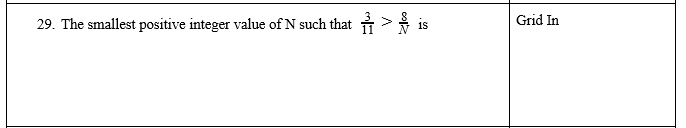Question #30: LCM of Three Numbers
1 point